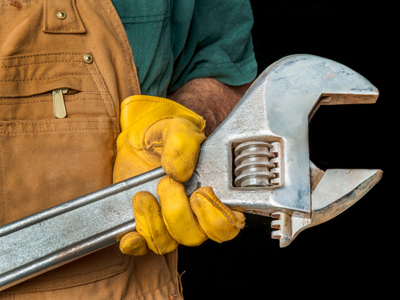Moments (turning forces) depend on force and distance from the pivot.

# Moments

This Science quiz is called 'Moments' and it has been written by teachers to help you if you are studying the subject at middle school. Playing educational quizzes is a fabulous way to learn if you are in the 6th, 7th or 8th grade - aged 11 to 14.

It costs only \$12.50 per month to play this quiz and over 3,500 others that help you with your school work. You can subscribe on the page at Join Us

Pushing or pulling the handle of a wrench connected to a nut or bolt produces a moment (turning force) that loosens or tightens the nut or bolt. In the field of Science, forces can be used to turn objects about a pivot (fixed point).

Take a moment or two to have a go at our quiz about moments!

1.
Moments (turning forces) depend on .......
force and distance from the pivot
force and mass
force and weight
mass and distance from the pivot
The unit for a moment is Nm - newtonmetre
2.
The moment will be increased .......
if the force and distance from the pivot are decreased
if the force and distance from the pivot are increased
if the force is decreased and distance is increased
if the force is increased and distance is decreased
Moment = force x distance
3.
Jane weighs 400N. She sits on a see-saw 1.5m from the pivot (center). Calculate the moment caused by Jane.
200Nm
400Nm
600Nm
800Nm
Moment = force x distance = 400 x 1.5 = 600Nm
4.
Amir weighs 500N. He sits on a see-saw 200cm from the pivot (center). Calculate the moment caused by Amir.
200Nm
700Nm
1,000Nm
10,000Nm
Distance = 200cm = 2m moment = 500 x 2 = 1,000Nm
5.
Two children weigh 300N and 400N, they sit 2m and 3m away from the pivot (center) on the same side of the see-saw. What is their total moment?
1,700Nm
1,800Nm
2,200Nm
3,500Nm
Moment = (300 x 2) + (400 x 3) = 1,800Nm
6.
A friend joins the two children (Q5) and sits on the other end of the see-saw. He weighs 600N. How far from the pivot must he sit to balance the see-saw?
2m
2.5m
3m
5m
Moment = force x distance, 1,800Nm = 600 x ? = 3m
7.
A spanner is 20cm long. A force of 10N is applied to undo a bolt. What is the moment (turning force)?
2Nm
20Nm
200Nm
2,000Nm
Distance = 20cm = 0. 2m moment = 10 x 0. 2 = 2Nm
8.
A 40cm spanner is used to produce the same turning force as Q7. What force would be needed?
Double the force
Quarter of the force
Half the force
The same force
9.
A moment of 50Nm is needed to open a jar. The pliers are 10cm long. What force is needed?
5N
50N
100N
500N
10cm = 0.1m, 50 = force x 0.1, force = 50/0.1 = 500N
10.
A moment of 80Nm is needed to undo a wheel nut. The spanner is 20cm long. What force is needed?
4N
80N
400N
800N
20cm = 0.2m, 80 = force x 0.2, force = 80/0.2 = 400N
Author:  Sue Davison